Symmetry of Crystals

The following article is from The Great Soviet Encyclopedia (1979). It might be outdated or ideologically biased.

Symmetry of Crystals

the ability of crystals to repeat themselves in the different positions under rotations, reflections, and parallel translations or combinations of these operations. The symmetry of the external form, that is, the faceting, of a crystal is determined by the symmetry of its atomic structure, which is also responsible for the symmetry of the physical properties of the crystal.

A quartz crystal is shown in Figure 1,a. Its external shape is such that under a 120° rotation about axis 3 the crystal will repeat itself (congruent equivalence). The crystal of sodium meta-silicate (Figure l,b) transforms into itself by reflection in the symmetry plane m (enantiomorphous equivalence). Thus, symmetry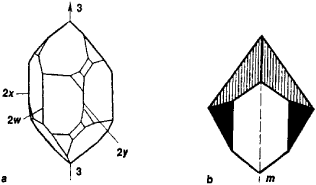Figure 1. Crystals of (a) quartz with a three-fold axis of symmetry 3 and the two-fold axes 2x, 2y, and 2w and (b) hydrated sodium meta-silicate with a symmetry plane m

means the possibility of transforming an object so that the object coincides with itself. If F(x1, x2, x3) is a function describing an object, for example, some property of a crystal or the shape of a crystal in three-dimensional space, and if the operation g[x1, x2, x3] accomplishes the transformation of the coordinates of all points of the object, then g is a symmetry operation, or transformation, and F is a symmetric object if the following conditions are satisfied: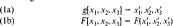In its most general formulation, symmetry is the invariance of objects under certain transformations of the variables describing the objects. Crystals are objects in three-dimensional space, and the classical theory of the symmetry of crystals is therefore a theory of symmetric transformations of three-dimensional space. The theory takes into account the three-dimensional translationally periodic pattern of the crystal’s internal structure, a structure described as a crystal lattice. Under symmetry transformations, space is not deformed but rather is transformed as a rigid whole (orthogonal, or isometric, transformation). After a symmetry transformation, the parts of an object located at one spot coincide with the parts located at another. This correspondence means that there are congruently or enantiomorphously equivalent parts in a symmetric object.

The symmetry of a crystal is manifested not only in the crystal’s structure and properties in real three-dimensional space but also in the description of the energy spectrum of the crystal’s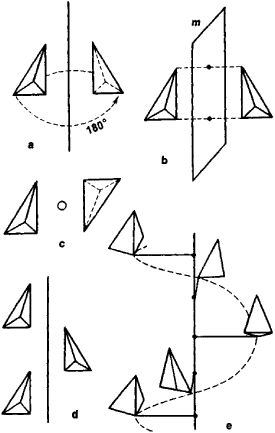Figure 2. The simplest symmetry operations: (a) rotation, (b) reflection, (c) inversion, (d) glide reflection, and (e) rotation about a fourfold screw axis

electrons in momentum space and in the analysis of the processes of X-ray diffraction in crystals by means of reciprocal space.

Symmetry group. More than one symmetry operation may be inherent in a crystal. For example, the quartz crystal in Figure 1,a transforms into itself not only under a 120° rotation about axis 3 (operation g,) but also under a 240° rotation about axis 3 (operation g2) and under a 180° rotation about the 2x, 2y, and 2w axes (operations g3, g4, and g5). For each symmetry operation there is a geometrical locus, referred to as a symmetry element (line, plane, point), with respect to which the given operation is performed. For example, the axis 3 or the axes 2x, 2y, and 2w are axes of symmetry, and the plane m (Figure 1,b) is a mirror plane of symmetry. The set of symmetry operations [g1, …, gn] of a given crystal forms a symmetry group G, in the sense of mathematical group theory. The performance of two symmetry operations in sequence also constitutes a symmetry operation. There always exists the identity operation g0 that changes nothing in the crystal and that corresponds geometrically to either a stationary condition or a 360° rotation about any axis. The number of operations of a group G is called the order of the group.

Symmetry groups can be classified according to the number n of dimensions of the space in which the groups are defined and the number m of dimensions of the space in which the object is periodic (designated, respectively, by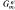). Various symmetry groups are used to describe crystals. Of these the most important are the space symmetry groups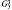, which describe the atomic structure of crystals, and the point symmetry groups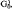, which describe the crystal’s external shape. The latter are also referred to as crystal classes.

Symmetry of crystal faces. The point symmetry operations include rotations by 360°/N about an N-fold axis of symmetry (Figure 2,a), reflection in a plane of symmetry (mirror reflection, Figure 2,b), inversion ī (symmetry with respect to a point, Figure 2,c), and rotary inversion (a combination of a 360°/N rotation with simultaneous inversion, Figure 2,d). Rotary reflection Ñ is sometimes used in place of rotary inversion in discussing symmetry. The geometrically possible combinations of these operations determine a particular point group (Figure 3) and are usually depicted in a stereographic projection. Under point symmetry transformations, at least one point of an object remains fixed; that is, the point is transformed into itself. All symmetry elements intersect at this point, and the point is the center of the stereographic projection.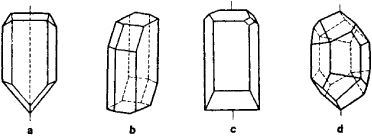Figure 3. Examples of crystals belonging to various point groups, or crystal classes: (a) class m (one plane of symmetry), (b) class c (one center of symmetry), (c) class 2 (one two-fold symmetry axis), and (d) class 6 (one six-fold axis of rotary reflection)

The point symmetry transformations g[x1 x2, x3] = x′1, x′2, x′3 are described by the linear eouations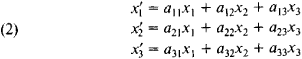that is, by the matrix of the coefficients (aij). For example, for a rotation about x3 by the angle a = 360°/N, the matrix of the coefficients has the form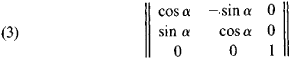Table 1. Symbols and names of the 32 point symmetry groups
SystemSymbolsNameRelations between unit cell constants
InternationalSchoenflies
Triclinic1C1Pedialabc
ciPinacoidalαβ ≠ γ ≠ 90°
Monoclinic2C2Sphenoidalabc
mCsDomaticα = γ = 90°
2/mC2hPrismaticβ90°
Orthorhombic222D2Rhombic-disphenoidalabc
mmC2vRhombic-pyramidalα = β = γ = 90°
mmmD2hRhombic-dipyramidal
Tetragonal4C4Tetragonal-pyramidala = b ≠c
422D4Tetragonal-trapezohedralα = β = γ = 90°
4/mC4hTetragonal-dipyramidal
4mmC4vDitetragonal-pyramidal
4/mmmD4hDitetragonal-dipyramidal
S4Tetragonal-disphenoidal
4̄2 mD2dTetragonal-scalenohedral
Trigonal3C3Trigonal-pyramidala = b = c
32D3Trigonal-trapezohedralα = β = y ≠ 90°
3mC3VDitrigonal-pyramidal
C3iRhombohedral
3̄mD3dHexagonal-scalenohedral
D3hTrigonal-dipyramidal
Hexagonal6̄2mD3hHexagonal-dipyramidala = b ≠ c
6C6Hexagonal-pyramidalα = β = 90°
62D6Hexagonal-trapezohedralγ = 120°
6/mC6hHexagonal-dipyramidal
6/mmC6vDihexagonal-pyramidal
6/mmmC6hDihexagonal-dipyramidal
Isometric23TTetartoidala = b = c
m3ThGyroidalα = β = γ = 90°
4̄3 mTdHextetrahedral
43ODiploidal
m3mOhHexoctahedral

For a reflection in the plane x1,, x2, the matrix has the form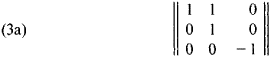Since N can have any value, the number of groupsis infinite. However, because of the lattices in crystals, only operations and, correspondingly, axes of symmetry up to six-fold (except for the five-fold axis) are permissible. These axes are designated by the symbols 1, 2, 3, 4, and 6. The possible axes of rotary inversion are designated by the symbols 1̄ (also a center of symmetry), 2̄ (equal to m, a plane of symmetry), 3̄, 4̄, and 6̄. The number of crystallographic point groups describing the external shape of crystals is therefore limited. These 32 crystal symmetry groups are set forth in Table 1. The international designations for the point groups include the symbols for the basic (generating) symmetry elements inherent in the groups. Depending on the symmetry of the unit cell (with lattice constants a, b, and c and angles α, β, and γ), the groups are combined in seven crystal systems: triclinic, monoclinic, orthorhombic, tetragonal, trigonal, hexagonal, and isometric. The proper classification of a crystal is determined by goniometry or X-ray diffraction analysis.

Groups containing only rotations describe crystals that consist solely of congruent parts. These groups are called groups of the first kind. Groups containing reflections or rotary inversions describe crystals in which there are enantiomor-phous parts (but there may also be congruent parts). These groups are called groups of the second kind. Crystals described by groups of the first kind can be crystallized in two enantio-morphous forms, by convention called right-handed and left-handed forms. Neither of the figures contains symmetry elements of the second kind, but the two are mirror images of each other.

Point groups describe the symmetry not only of crystals but of any finite figure. In the plant and animal world, symmetry is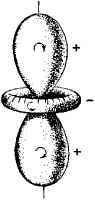Figure 4. Surface describing the optical activity of a quartz crystal in which the signs + and — indicate opposite directions of rotation of the plane of polarization

often observed with five-fold and seven-fold axes and axes of even higher orders, symmetry that is forbidden in crystallography. For example, the icosahedral point group 532 has turned out to be important for describing the regular structure of spherical viruses in whose shells the crystallographic principles of dense molecular packing are observed.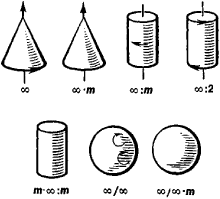Figure 5. Figures illustrating limit groups of symmetry

Symmetry of physical properties; limit groups. With regard to macroscopic physical properties (optical, electrical, mechanical), crystals behave like a homogeneous anisotropic medium; that is, the discreteness of their atomic structure is not manifested. Homogeneity means that the properties are identical at any point in the crystal; however, at the same time many properties depend on direction. The dependence on direction can be represented as a function, and a reference surface of a given property can be constructed (Figure 4). This function, which may be different for different physical properties of the crystal (vector or tensor), has a definite point symmetry directly related to the symmetry group of the crystal faces. This point symmetry is either the same as or higher than the symmetry of the crystal faces (Neumann’s principle).

Many of the properties of crystals that belong to certain classes are described by limit point groups containing symmetry axes of infinite order, designated by oo. The existence of an oo-fold axis means that the object is superposed upon itself under a rotation of any angle, including an infinitely small one. There are seven such groups; they are represented in Figure 5 by sample figures and corresponding symbols. In all, there are thus 32 + 7 = 39 point groups describing the symmetry of crystal properties. If the symmetry group of a crystal is known, the possibility of the presence or absence of certain physical properties can be indicated.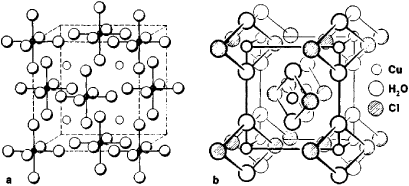Figure 6. Unit cells of crystals of (a) K2 PtCI6 and (b) CuCI2·2H2 O

Space symmetry of the atomic structure of crystals (crystal lattice). Space symmetry is described by the space symmetry groups. Three noncoplanar translations, a, b, and c, are characteristic operations for the lattice. The translations give the three-dimensional periodicity of the atomic structure of crystals. Displacement of the structure by the vectors a1, b2, c3 or by any vector t = p1a1 + p2b2 + p3c3, where p1, p2, and p3 are any positive or negative whole number, superposes the structure of the crystal upon itself and consequently is a symmetry operation that satisfies the conditions of equations (1a) and (1b). The parallelepiped constructed on vectors a, b, and c is called the unit cell of the crystal (Figure 6). The unit cell contains some minimum grouping of atoms the repetition of which by symmetry operations, including translation, forms the crystal lattice. The unit cell and the arrangement of atoms therein are determined by the techniques of X-ray diffraction analysis, electron diffraction, or neutron diffraction.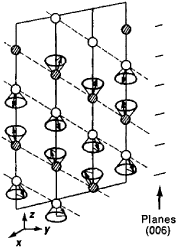Figure 7. Distribution of magnetic moments (arrows) in the unit cell of a Cr2 O3 crystal

As a result of the possibility of combining translations and point symmetry operations in the lattice ingroups, symmetry operations and corresponding symmetry elements arise with a translational component— screw axes of various orders and glide reflection planes (Figure 2,e).

In all, 230 space (Fedorov) symmetry groupsare known, and every crystal belongs to one of these groups. The translational components of microsymmetry elements are not manifested macroscopically; for example, the screw axis is manifested in the crystal faces as a simple axis of rotation of the corresponding order. Each of the 230groups is therefore macroscopically similar to one of the 32 point groups. For example, 28 space groups are compatible with the point group mmm, or D2h. The set of translations inherent in a given space group is the translational subgroup, or Bravais lattice; there are 14 such lattices.

Symmetry of layers and chains.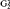groups— two-dimensional periodic groups—and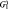groups—one-dimensional periodic groups in three-dimensional space—may be used to describe planar or unidirectionally extended fragments of the crystal structure. These groups play an important role in the study of biological structures and molecules. For example,groups describe the structure of biological membranes, andgroups, the structure of the chain molecules of rod-shaped viruses and tubular crystals of globular proteins in which the molecules are laid out in the spiral (screw) symmetry that is possible ingroups.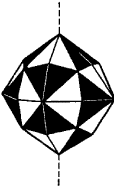Figure 8. Figure described by an antisymmetry point group

Generalized symmetry. The concept of equivalence under transformation, expressed in equations (la) and (lb), underlies the definition of symmetry. However, physically (and mathematically) an object can be equivalent to itself in certain characteristics and not equivalent in others. For example, the distribution of nuclei and electrons in the crystal of an antiferromagnetic substance can be described using ordinary space symmetry. If, however, the distribution of magnetic moments in the crystal (Figure 7) is taken into account, “ordinary” classical symmetry is no longer adequate. Antisymmetry and color symmetry are among such generalizations of symmetry. In antisymmetry, in addition to the three space variables x1, x2, and x3, a fourth variable x4 = ± 1 is introduced. This may be interpreted in such a way that under the transformation of equation (1) function F not only can be equivalent to itself, as in equation (1b) but also can be of opposite sign. Such an operation can be represented by a change in color (Figure 8). There are 58 point antisymmetry groups G03,a and 1,651 space antisymmetry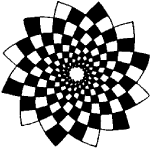Figure 9. An example of similarity symmetry

groups G33,a (Shubnikov groups). If the additional variable assumes not two values but several (the numbers 3, 4, 6, 8, …, 48 are possible), then Belov’s “color” symmetry arises. Thus, 81 point groups G03,u are known. The main applications of generalized symmetry in crystallography are in describing magnetic structures.

Other generalizations of symmetry include (1) similarity symmetry, in which equivalence of parts of a figure is replaced by similarity (Figure 9), (2) curvilinear symmetry, and (3) statistical symmetry, which is introduced in describing the structure of disordered crystals, solid solutions, and liquid crystals.

REFERENCES

Shubnikov, A. V., and V. A. Koptsik. Simmetriia v nauke i iskusstve, 2nd ed. Moscow, 1972.
Weyl, H. Simmetriia. Moscow, 1968. (Translated from English.)
Fedorov, E. S. Simmetriia i struktura kristallov. [Moscow] 1949.
Shubnikov, A. V. Simmetriia i antisimmetriia konechnykh figur. Moscow, 1951.

B. K. VAINSHTEIN